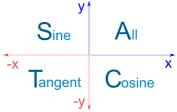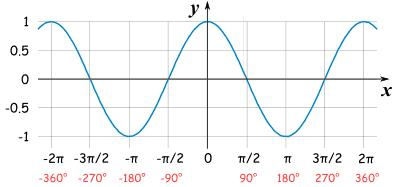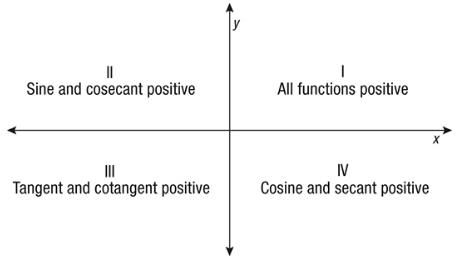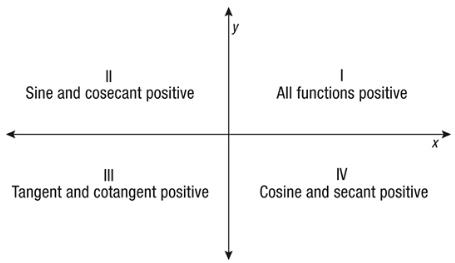Courses

# Test: Introduction To Trigonometric Functions

## 10 Questions MCQ Test Mathematics (Maths) Class 11 | Test: Introduction To Trigonometric Functions

Description
This mock test of Test: Introduction To Trigonometric Functions for JEE helps you for every JEE entrance exam. This contains 10 Multiple Choice Questions for JEE Test: Introduction To Trigonometric Functions (mcq) to study with solutions a complete question bank. The solved questions answers in this Test: Introduction To Trigonometric Functions quiz give you a good mix of easy questions and tough questions. JEE students definitely take this Test: Introduction To Trigonometric Functions exercise for a better result in the exam. You can find other Test: Introduction To Trigonometric Functions extra questions, long questions & short questions for JEE on EduRev as well by searching above.
QUESTION: 1

### What is the value of cos x in second quadrant if sin x = 3/5 in II quadrant

Solution:

We know that
sinx = p÷h
now p= 3 & h=5 ,
so b = sq. root of 5^2 - 3^2= 4
So Cosx= b÷h = 4÷5
but in 2nd quad. cos is -ve ,
therefore Cosx = -4÷5

QUESTION: 2

### What is the value of cos 41π/4

Solution:

We know ,π = 180deg

So  cos 41π/4 = Cos( 41*180/4)

= Cos (1845deg)

= Cos (1800 + 45)

= Cos (10π + π/4)

= Cos (π/4)

= 1/√2

QUESTION: 3

### In which quadrant are sin, cos and tan positive?

Solution:
• All three of them are positive in Quadrant I
• Sine only is positive in Quadrant II
• Tangent only is positive in Quadrant III
• Cosine only is positive in Quadrant IV
•QUESTION: 4

What is the range of cos function?

Solution:

Just look at the graph of cosine.We know , Range of a function is the set of all possible outputs for that function. If you look at any 2π interval, the cosine function is periodic after every 2π.  So th range for cos function is [-1,1]

QUESTION: 5

What is the value of sin 7π ?

Solution:

Sin 7π = Sin 7*180 = Sin 2π * 7  = 0

QUESTION: 6

Which of the following cannot be the value of cos θ.

Solution:

√2 cannot be the value for Cosθ.

The values of  Cos θ at different angles are given below :

Cos0°=1

Cos30°=√3/2

Cos45°=1/√2

Cos60°=1/2

Cos90°=0

QUESTION: 7

What is the sign of the sinA and tanA in third quadrant respectively

Solution:QUESTION: 8

tan x = - 5/12, x lies in the second quadrant. So sinx = ?

Solution:
QUESTION: 9

What is the sign of the sec θ and cosec θ in second quadrant respectively?

Solution:

In quadrant sin, cos tan, cot, sec, cosec all +ve .In second quadrant sin and cosec are +ve. in 3rd quadrant tan and cot are positive.And in 4th cos and sec are +ve.QUESTION: 10

Identify the odd one out from the following

Solution: